Aldol condensation will not take place in            

(a) HCHO                       (b) CH3CHO

(c) CH3COCH3                (d) CH3CH2CHO

Concept Questions :-

Preparation & properties of Aldehydes and Ketones
High Yielding Test Series + Question Bank - NEET 2020

Difficulty Level:

A and B in the following reactions are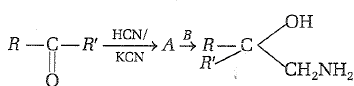(a) A=RR'CH2CN,              B=NaOH

(b) A=RR'C${<}_{\mathrm{COOH}}^{\mathrm{OH}}$ ,          B=CH3

(c) A=RR'C${<}_{\mathrm{COOH}}^{\mathrm{CN}}$ ,          B=CH3

(d) A=RR"C${<}_{\mathrm{OH}}^{\mathrm{CN}}$,              B=LiAlH4

Concept Questions :-

Preparation & properties of Aldehydes and Ketones
High Yielding Test Series + Question Bank - NEET 2020

Difficulty Level:

RCOOH $\to$RCH2OH. This mode of reduction of an acid to alcohol can be affected only by:

1. Zn/HCl

2. Na-alcohol

3. aluminium isopropoxide and isopropyl alcohol

4. LiAlH4

Concept Questions :-

Preparation & properties of Aldehydes and Ketones
High Yielding Test Series + Question Bank - NEET 2020

Difficulty Level:

Ethyl ester $\underset{\mathrm{Excess}}{\overset{{\mathrm{CH}}_{3}\mathrm{MgBr}}{\to }}$ P. the product P will be:

1.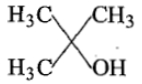2.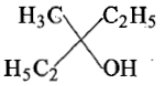3.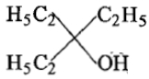4.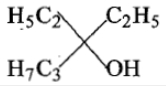Concept Questions :-

Preparation & properties of Aldehydes and Ketones
High Yielding Test Series + Question Bank - NEET 2020

Difficulty Level:

Which of the following has most acidic proton?

(a) CH3COCH3                             (b) (CH3)2C=CH2

(c) CH3COCH2COCH3                   (d) (CH3.CO)3CH

Concept Questions :-

Preparation & properties of Aldehydes and Ketones
High Yielding Test Series + Question Bank - NEET 2020

Difficulty Level:

Which one of the following can be oxidised to the corresponding carbonyl compound?   

(a) 2-hydroxy propane

(b) Ortho-nitro phenol

(c) Phenol

(d) 2-methyl-2-hydroxy propane

Concept Questions :-

Preparation & properties of Aldehydes and Ketones
High Yielding Test Series + Question Bank - NEET 2020

Difficulty Level:

Dry distillation of barium salt of Hexane-1,2-dicarboxylic acid gives: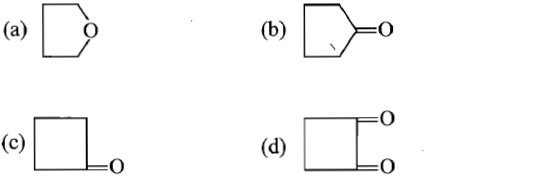Concept Questions :-

Preparation & properties of Aldehydes and Ketones
High Yielding Test Series + Question Bank - NEET 2020

Difficulty Level:

Polarisation of electrons in acrolein may be written as

1. $\stackrel{\mathrm{\delta }+}{\mathrm{C}}{\mathrm{H}}_{2}=\mathrm{CH}-\mathrm{CH}=\stackrel{\mathrm{\delta }-}{\mathrm{O}}$

2. $\stackrel{\mathrm{\delta }+}{\mathrm{C}}{\mathrm{H}}_{2}=\stackrel{\mathrm{\delta }+}{\mathrm{C}}\mathrm{H}-\mathrm{CH}=\stackrel{}{\mathrm{O}}$

3. $\stackrel{\mathrm{\delta }+}{\mathrm{C}}{\mathrm{H}}_{2}=\stackrel{}{\mathrm{C}}\mathrm{H}-\mathrm{CH}=\stackrel{\delta +}{\mathrm{O}}$

4. $\stackrel{\mathrm{\delta }+}{\mathrm{C}}{\mathrm{H}}_{2}=\mathrm{CH}-\stackrel{\mathrm{\delta }+}{\mathrm{C}}\mathrm{H}=\mathrm{O}$

Concept Questions :-

Preparation & properties of Aldehydes and Ketones
High Yielding Test Series + Question Bank - NEET 2020

Difficulty Level:

Consider the following reaction;

CH3Br + Mg $\stackrel{\mathrm{Ether}}{\to }$A$\stackrel{\mathrm{HCHO}}{\to }$B$\stackrel{\mathrm{HOH}}{\to }$C

compound C is:

(a) acetic acid                              (b) acetaldehyde

(c) ethyl alcohol                            (d) formic acid

Concept Questions :-

Preparation & properties of Aldehydes and Ketones
High Yielding Test Series + Question Bank - NEET 2020

Difficulty Level:

The conversion of CH3OH to CH3COOH can be brought in by:

1. K2Cr2O7/H+

2. CO + Rh

3. KMnO4

4. H3PO4

Concept Questions :-

Carboxylic Acids